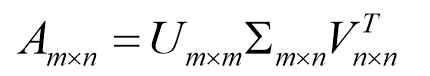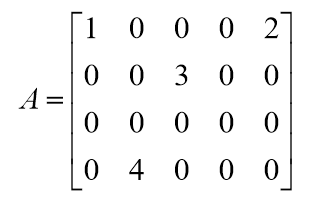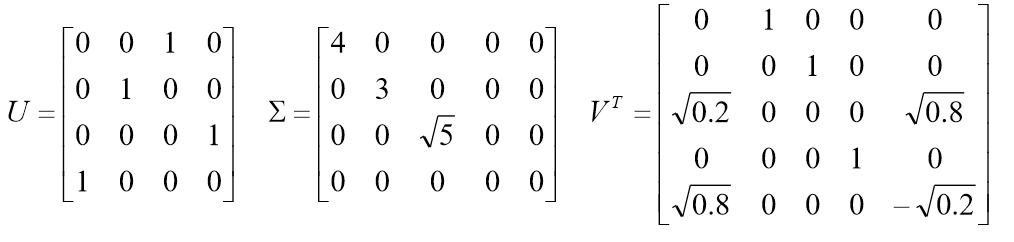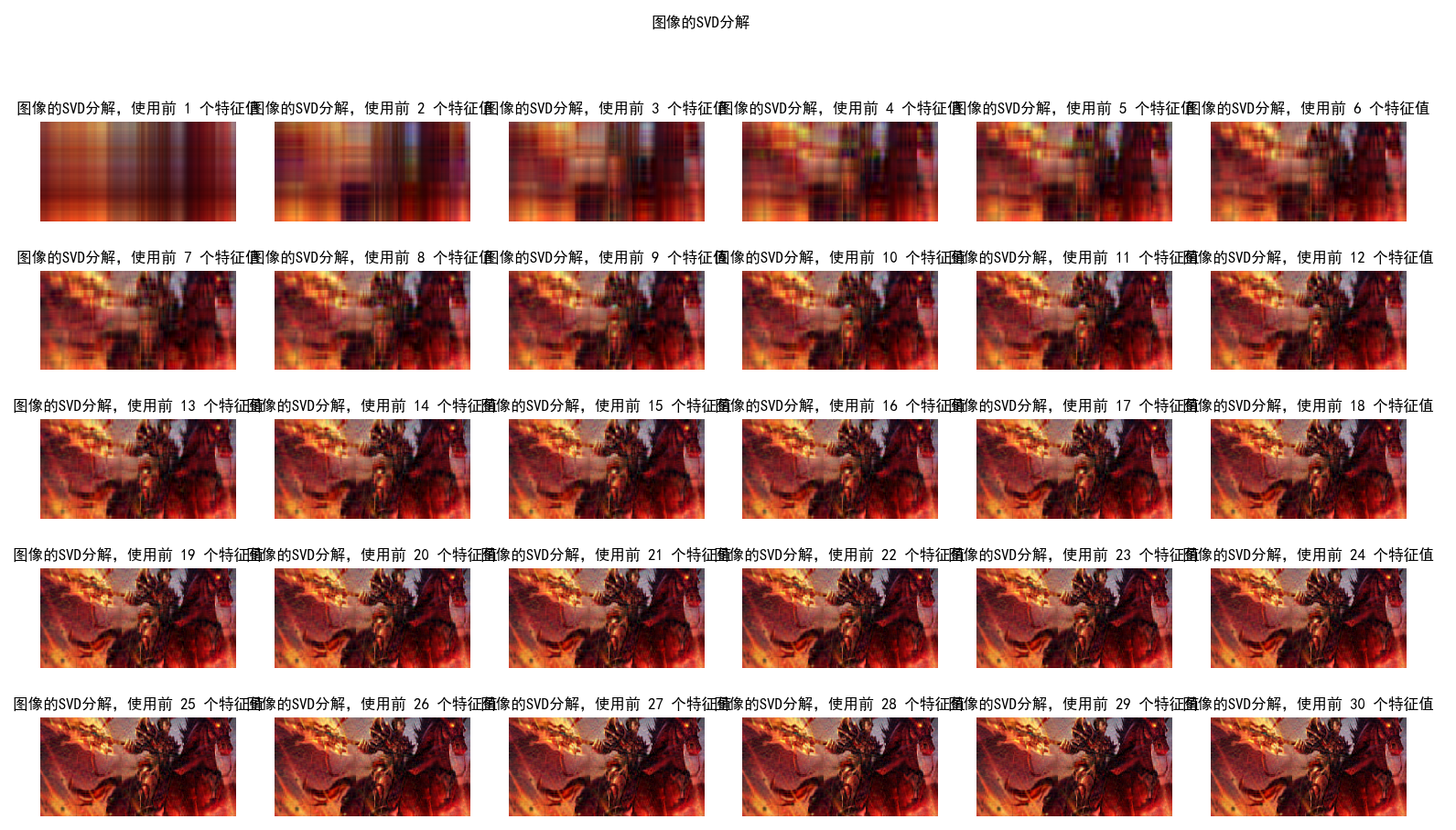# python——矩阵的奇异值分解，对图像进行SVD

奇异值分解(Singular Value Decomposition)是一种重要的矩阵分解方法，可以看做是对方阵在任意矩阵上的推广。Singular的意思是突出的，奇特的，非凡的，按照这样的翻译似乎也可以叫做矩阵的优值分解。

假设矩阵A是一个m*n阶的实矩阵，则存在一个分解使得：下面给出一个实际的例子，对矩阵A进行奇异值分解：矩阵奇异值分解的运用非常的广泛，PCA，推荐系统，数据压缩，矩阵分解，这里就不介绍它的推导过程和原理了，想了解的同学可以查阅相关的资料，下面我们使用SVD来对图像进行分解，使用不同数量的奇异值来对图像进行压缩。我们的图像是500*980大小，总得奇异值有500个，当我们使用30个奇异值的时候，发现图像已经有点清晰了，确实很强大。

import numpy as np
import matplotlib.image as mping
import matplotlib.pyplot as plt
import matplotlib as mpl

def image_svd(n, pic):
a, b, c = np.linalg.svd(pic)
svd = np.zeros((a.shape,c.shape))
for i in range(0, n):
svd[i, i] = b[i]
img = np.matmul(a, svd)
img = np.matmul(img, c)
img[ img >= 255] = 255
img[  0 >= img ] = 0
img = img.astype(np.uint8)
return img

if __name__ == '__main__':
mpl.rcParams['font.sans-serif'] = ['SimHei']
mpl.rcParams['axes.unicode_minus'] = False

path = './simplepython/ImgSVD/a.jpg'
print(img.shape)

r = img[:, :, 0]
g = img[:, :, 1]
b = img[:, :, 2]
plt.figure(figsize=(50, 100))
for i in range(1, 31):
r_img = image_svd(i, r)
g_img = image_svd(i, g)
b_img = image_svd(i, b)
pic = np.stack([r_img, g_img, b_img], axis=2)
print(i)
plt.subplot(5, 6, i)
plt.title("图像的SVD分解，使用前 %d 个特征值" %(i))
plt.axis('off')
plt.imshow(pic)
plt.suptitle("图像的SVD分解")
plt.show()